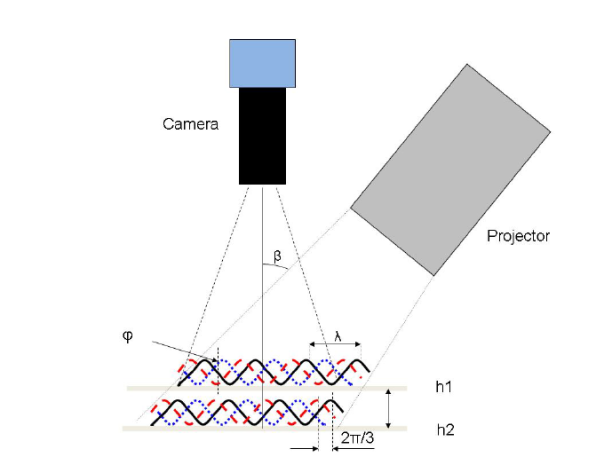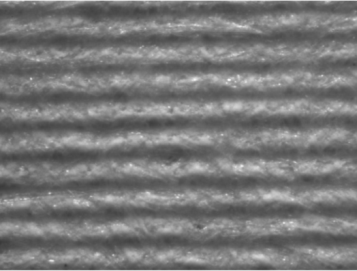# Corrected contact angle

##### Corrected contact angle – How topography in real-life samples can affect your measurements

Contact angle measurements are widely used in research, production, and quality control fields. Background on the theory and significance of these measurements is provided on the contact angle goniometry – tensiometry pages. The Young’s contact angle of a material, which is usually the value reported in literature, assumes an ideal surface that contains no roughness, contaminants, chemical heterogeneity, or interactions with the probe liquid. In most real-life situations, one or more of these assumptions may be violated. In particular, roughness has a well-known effect on contact angle, where increasing amounts of roughness tend to enhance the existing properties of the material. For research in understanding the fundamental properties of a coating, plastic or ceramic, these roughness effects may be unwanted and could paint an inaccurate picture of the true material properties.

In 1936, Robert Wenzel developed a relationship between the Young’s contact angle, surface roughness, and the corrected contact angle :(1)

where θm is the measured contact angle, θY is the Young’s contact angle, and r is:(2)

The Sdr is defined as the additional percentage in surface area contributed by surface roughness and can be measured using a device such as a profilometer or atomic force microscope. The Sdr of a completely flat surface is 0. Its calculated value per ISO 25178  is:(3)

where A is the definition area.

An integrated system that contains both a contact angle goniometer and a profilometer could potentially perform both a Sdr measurement and contact angle on the exact same spot on a sample. The Theta Flex with Topography is one such instrument. By using an automated XYZ stage, the instrument can accurately move between where the topography measurement is performed and where the contact angle droplet is placed.Figure 1 – The Theta Flex with Topography integrated contact angle and topography measurement system

The Topography Module utilizes a fringe projection phase shifting method where a projector generates a sinusoidal fringe pattern on a sample and the movement of the fringes is captured with a camera. The instrument setup is shown in Figure 2. In this system, the sinusoidal fringes can be described by the equation:(4)

Where x and y are the position coordinate, a is the background intensity, b is the amplitude modulation, p is the sinusoidal grating wavelength, ϕ0 is the phase shift due to the surface height, and δn is the phase shift from the slide movement. The intensity of each pixel varies as a function of height, and using a software algorithm, topographical information such as the Sdr, root means squared (RMS) roughness, average height, minimum and maximum height, and other parameters can be determined in 2D and 3D. More details about the fringe projection phase shifting method can be found from Zhang and Peisen .Figure 2 – Schematic of a fringe-projection phase shifting measurement and its corresponding optical surface

Once the Sdr of the surface has been measured, the corrected contact angle, i.e. the Young’s contact angle θY can be determined through equations (1) and (2). This allows one to determine the contact angle as well as associated information such as the surface free energy of a perfectly flat sample. Such information is critical for a fundamental understanding of materials and coatings.

References: